Paper Cutting Quiz II, Non Verbal Reasoning

# Paper Cutting Quiz II, Non Verbal Reasoning - Mechanical Engineering

Test Description

## 25 Questions MCQ Test General Aptitude for GATE - Paper Cutting Quiz II, Non Verbal Reasoning

Paper Cutting Quiz II, Non Verbal Reasoning for Mechanical Engineering 2023 is part of General Aptitude for GATE preparation. The Paper Cutting Quiz II, Non Verbal Reasoning questions and answers have been prepared according to the Mechanical Engineering exam syllabus.The Paper Cutting Quiz II, Non Verbal Reasoning MCQs are made for Mechanical Engineering 2023 Exam. Find important definitions, questions, notes, meanings, examples, exercises, MCQs and online tests for Paper Cutting Quiz II, Non Verbal Reasoning below.
Solutions of Paper Cutting Quiz II, Non Verbal Reasoning questions in English are available as part of our General Aptitude for GATE for Mechanical Engineering & Paper Cutting Quiz II, Non Verbal Reasoning solutions in Hindi for General Aptitude for GATE course. Download more important topics, notes, lectures and mock test series for Mechanical Engineering Exam by signing up for free. Attempt Paper Cutting Quiz II, Non Verbal Reasoning | 25 questions in 50 minutes | Mock test for Mechanical Engineering preparation | Free important questions MCQ to study General Aptitude for GATE for Mechanical Engineering Exam | Download free PDF with solutions
 1 Crore+ students have signed up on EduRev. Have you?
Paper Cutting Quiz II, Non Verbal Reasoning - Question 1

### Directions to Solve Each of the following questions consists of a set of three figures X, Y and Z showing a sequence of folding of apiece of paper. Figure (Z) shows the manner in which the folded paper has been cut. These three figures are followed by four answer figures from which you have to choose a figure which would most closely resemble the unfolded form of figure (Z). Question - Choose a figure which would most closely resemble the unfolded form of Figure (Z).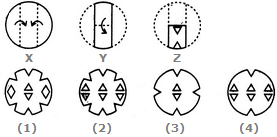Paper Cutting Quiz II, Non Verbal Reasoning - Question 2

### Directions to Solve Each of the following questions consists of a set of three figures X, Y and Z showing a sequence of folding of apiece of paper. Figure (Z) shows the manner in which the folded paper has been cut. These three figures are followed by four answer figures from which you have to choose a figure which would most closely resemble the unfolded form of figure (Z). Question - Choose a figure which would most closely resemble the unfolded form of Figure (Z).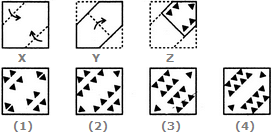Paper Cutting Quiz II, Non Verbal Reasoning - Question 3

### Directions to Solve Each of the following questions consists of a set of three figures X, Y and Z showing a sequence of folding of apiece of paper. Figure (Z) shows the manner in which the folded paper has been cut. These three figures are followed by four answer figures from which you have to choose a figure which would most closely resemble the unfolded form of figure (Z). Question - Choose a figure which would most closely resemble the unfolded form of Figure (Z).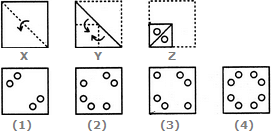Paper Cutting Quiz II, Non Verbal Reasoning - Question 4

Each of the following questions consists of a set of three figures X, Y and Z showing a sequence of folding of apiece of paper. Figure (Z) shows the manner in which the folded paper has been cut. These three figures are followed by four answer figures from which you have to choose a figure which would most closely resemble the unfolded form of figure (Z).

Question -

Choose a figure which would most closely resemble the unfolded form of Figure (Z).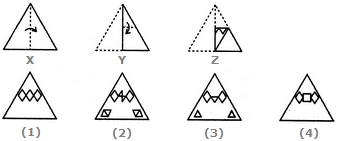Paper Cutting Quiz II, Non Verbal Reasoning - Question 5

Each of the following questions consists of a set of three figures X, Y and Z showing a sequence of folding of apiece of paper. Figure (Z) shows the manner in which the folded paper has been cut. These three figures are followed by four answer figures from which you have to choose a figure which would most closely resemble the unfolded form of figure (Z).

Question -

Choose a figure which would most closely resemble the unfolded form of Figure (Z).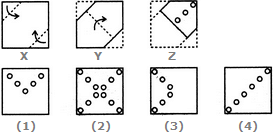Paper Cutting Quiz II, Non Verbal Reasoning - Question 6

Each of the following questions consists of a set of three figures X, Y and Z showing a sequence of folding of apiece of paper. Figure (Z) shows the manner in which the folded paper has been cut. These three figures are followed by four answer figures from which you have to choose a figure which would most closely resemble the unfolded form of figure (Z).

Question -

Choose a figure which would most closely resemble the unfolded form of Figure (Z).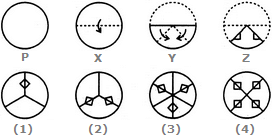Paper Cutting Quiz II, Non Verbal Reasoning - Question 7

Each of the following questions consists of a set of three figures X, Y and Z showing a sequence of folding of apiece of paper. Figure (Z) shows the manner in which the folded paper has been cut. These three figures are followed by four answer figures from which you have to choose a figure which would most closely resemble the unfolded form of figure (Z).

Question -

Choose a figure which would most closely resemble the unfolded form of Figure (Z).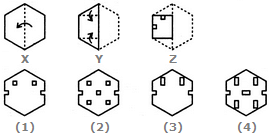Paper Cutting Quiz II, Non Verbal Reasoning - Question 8

Each of the following questions consists of a set of three figures X, Y and Z showing a sequence of folding of apiece of paper. Figure (Z) shows the manner in which the folded paper has been cut. These three figures are followed by four answer figures from which you have to choose a figure which would most closely resemble the unfolded form of figure (Z).

Question -

Choose a figure which would most closely resemble the unfolded form of Figure (Z).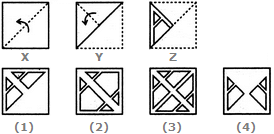Paper Cutting Quiz II, Non Verbal Reasoning - Question 9

Each of the following questions consists of a set of three figures X, Y and Z showing a sequence of folding of apiece of paper. Figure (Z) shows the manner in which the folded paper has been cut. These three figures are followed by four answer figures from which you have to choose a figure which would most closely resemble the unfolded form of figure (Z).

Question -

Choose a figure which would most closely resemble the unfolded form of Figure (Z).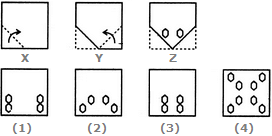Paper Cutting Quiz II, Non Verbal Reasoning - Question 10

Each of the following questions consists of a set of three figures X, Y and Z showing a sequence of folding of apiece of paper. Figure (Z) shows the manner in which the folded paper has been cut. These three figures are followed by four answer figures from which you have to choose a figure which would most closely resemble the unfolded form of figure (Z).

Question -

Choose a figure which would most closely resemble the unfolded form of Figure (Z).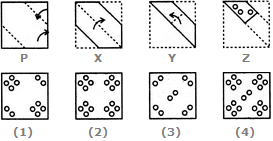Paper Cutting Quiz II, Non Verbal Reasoning - Question 11

Each of the following questions consists of a set of three figures X, Y and Z showing a sequence of folding of apiece of paper. Figure (Z) shows the manner in which the folded paper has been cut. These three figures are followed by four answer figures from which you have to choose a figure which would most closely resemble the unfolded form of figure (Z).

Question -

Choose a figure which would most closely resemble the unfolded form of Figure (Z).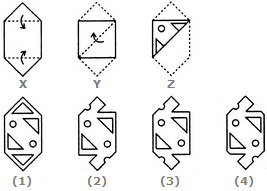Paper Cutting Quiz II, Non Verbal Reasoning - Question 12

Each of the following questions consists of a set of three figures X, Y and Z showing a sequence of folding of apiece of paper. Figure (Z) shows the manner in which the folded paper has been cut. These three figures are followed by four answer figures from which you have to choose a figure which would most closely resemble the unfolded form of figure (Z).

Question -

Choose a figure which would most closely resemble the unfolded form of Figure (Z).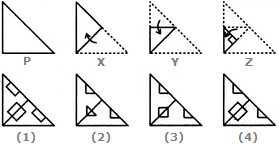Paper Cutting Quiz II, Non Verbal Reasoning - Question 13

Each of the following questions consists of a set of three figures X, Y and Z showing a sequence of folding of apiece of paper. Figure (Z) shows the manner in which the folded paper has been cut. These three figures are followed by four answer figures from which you have to choose a figure which would most closely resemble the unfolded form of figure (Z).

Question -

Choose a figure which would most closely resemble the unfolded form of Figure (Z).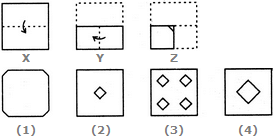Paper Cutting Quiz II, Non Verbal Reasoning - Question 14

Each of the following questions consists of a set of three figures X, Y and Z showing a sequence of folding of apiece of paper. Figure (Z) shows the manner in which the folded paper has been cut. These three figures are followed by four answer figures from which you have to choose a figure which would most closely resemble the unfolded form of figure (Z).

Question -

Choose a figure which would most closely resemble the unfolded form of Figure (Z).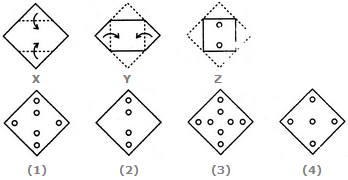Paper Cutting Quiz II, Non Verbal Reasoning - Question 15

Each of the following questions consists of a set of three figures X, Y and Z showing a sequence of folding of apiece of paper. Figure (Z) shows the manner in which the folded paper has been cut. These three figures are followed by four answer figures from which you have to choose a figure which would most closely resemble the unfolded form of figure (Z).

Question -

Choose a figure which would most closely resemble the unfolded form of Figure (Z).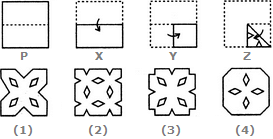Paper Cutting Quiz II, Non Verbal Reasoning - Question 16

Each of the following questions consists of a set of three figures X, Y and Z showing a sequence of folding of apiece of paper. Figure (Z) shows the manner in which the folded paper has been cut. These three figures are followed by four answer figures from which you have to choose a figure which would most closely resemble the unfolded form of figure (Z).

Question -

Choose a figure which would most closely resemble the unfolded form of Figure (Z).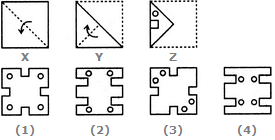Paper Cutting Quiz II, Non Verbal Reasoning - Question 17

Each of the following questions consists of a set of three figures X, Y and Z showing a sequence of folding of apiece of paper. Figure (Z) shows the manner in which the folded paper has been cut. These three figures are followed by four answer figures from which you have to choose a figure which would most closely resemble the unfolded form of figure (Z).

Question -

Choose a figure which would most closely resemble the unfolded form of Figure (Z).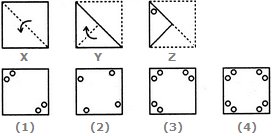Paper Cutting Quiz II, Non Verbal Reasoning - Question 18

Each of the following questions consists of a set of three figures X, Y and Z showing a sequence of folding of apiece of paper. Figure (Z) shows the manner in which the folded paper has been cut. These three figures are followed by four answer figures from which you have to choose a figure which would most closely resemble the unfolded form of figure (Z).

Question -

Choose a figure which would most closely resemble the unfolded form of Figure (Z).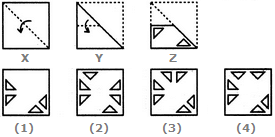Paper Cutting Quiz II, Non Verbal Reasoning - Question 19

Each of the following questions consists of a set of three figures X, Y and Z showing a sequence of folding of apiece of paper. Figure (Z) shows the manner in which the folded paper has been cut. These three figures are followed by four answer figures from which you have to choose a figure which would most closely resemble the unfolded form of figure (Z).

Question -

Choose a figure which would most closely resemble the unfolded form of Figure (Z).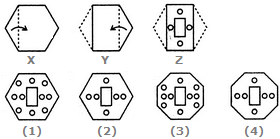Paper Cutting Quiz II, Non Verbal Reasoning - Question 20

Each of the following questions consists of a set of three figures X, Y and Z showing a sequence of folding of apiece of paper. Figure (Z) shows the manner in which the folded paper has been cut. These three figures are followed by four answer figures from which you have to choose a figure which would most closely resemble the unfolded form of figure (Z).

Question -

Choose a figure which would most closely resemble the unfolded form of Figure (Z).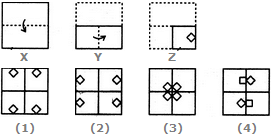Paper Cutting Quiz II, Non Verbal Reasoning - Question 21

Each of the following questions consists of a set of three figures X, Y and Z showing a sequence of folding of apiece of paper. Figure (Z) shows the manner in which the folded paper has been cut. These three figures are followed by four answer figures from which you have to choose a figure which would most closely resemble the unfolded form of figure (Z).

Question -

Choose a figure which would most closely resemble the unfolded form of Figure (Z).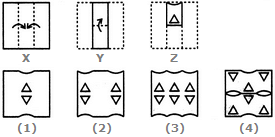Paper Cutting Quiz II, Non Verbal Reasoning - Question 22

Each of the following questions consists of a set of three figures X, Y and Z showing a sequence of folding of apiece of paper. Figure (Z) shows the manner in which the folded paper has been cut. These three figures are followed by four answer figures from which you have to choose a figure which would most closely resemble the unfolded form of figure (Z).

Question -

Choose a figure which would most closely resemble the unfolded form of Figure (Z).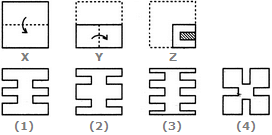Paper Cutting Quiz II, Non Verbal Reasoning - Question 23

Each of the following questions consists of a set of three figures X, Y and Z showing a sequence of folding of apiece of paper. Figure (Z) shows the manner in which the folded paper has been cut. These three figures are followed by four answer figures from which you have to choose a figure which would most closely resemble the unfolded form of figure (Z).

Question -

Choose a figure which would most closely resemble the unfolded form of Figure (Z).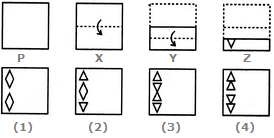Paper Cutting Quiz II, Non Verbal Reasoning - Question 24

Each of the following questions consists of a set of three figures X, Y and Z showing a sequence of folding of apiece of paper. Figure (Z) shows the manner in which the folded paper has been cut. These three figures are followed by four answer figures from which you have to choose a figure which would most closely resemble the unfolded form of figure (Z).

Question -

Choose a figure which would most closely resemble the unfolded form of Figure (Z).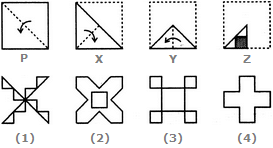Paper Cutting Quiz II, Non Verbal Reasoning - Question 25

25.Choose a figure which would most closely resemble the unfolded form of Figure (Z).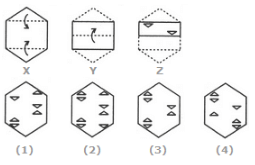## General Aptitude for GATE

84 videos|84 docs|108 tests
Information about Paper Cutting Quiz II, Non Verbal Reasoning Page
In this test you can find the Exam questions for Paper Cutting Quiz II, Non Verbal Reasoning solved & explained in the simplest way possible. Besides giving Questions and answers for Paper Cutting Quiz II, Non Verbal Reasoning, EduRev gives you an ample number of Online tests for practice

## General Aptitude for GATE

84 videos|84 docs|108 tests